Documentation

Model and Validate a System

You model each component within the system structure to represent the physical or functional behavior of that component. You verify the basic component behavior by simulating them using test data.

Open the System Layout

A big-picture view of the whole system layout is useful when modeling individual components. Start by loading the layout model. At the MATLAB command line, enter:

open_system('system_layout.slx')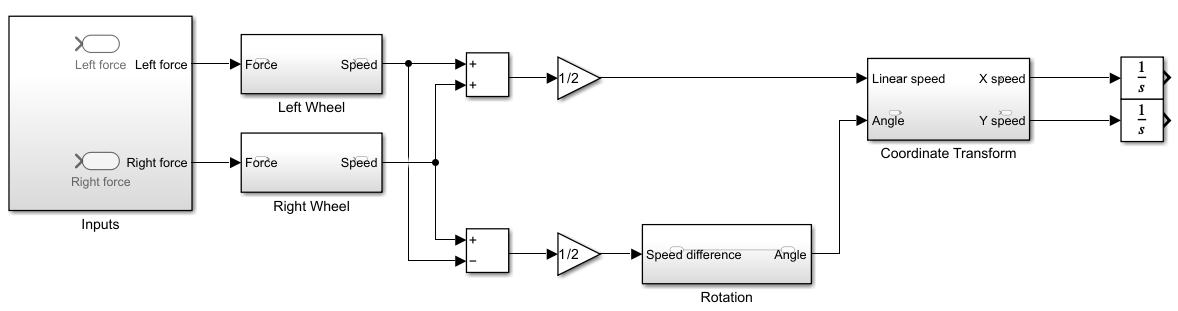Model the Components

A Simulink® model of a component is based on several starting points:

• An explicit mathematical relationship between the output and the input of a physical component — You can compute the outputs of the component from the inputs, directly or indirectly, through algebraic computations and integration of differential equations. For example, computation of the water level in a tank given the inflow rate is an explicit relationship. Each Simulink block executes based on the definition of the computations from its inputs to its outputs.

• An implicit mathematical relationship between model variables of a physical component — Because variables are interdependent, assigning an input and an output to the component is not straightforward. For example, the voltage at the + end of a motor connected in a circuit and the voltage at the - end have an implicit relationship. To model such a relationship in Simulink, you can either use physical modeling tools such as Simscape™ or model these variables as part of a larger component that allows input/output definition. Sometimes, closer inspection of modeling goals and component definitions helps to define input/output relationships.

• Data obtained from an actual system — You have measured input/output data from the actual component, but a fully defined mathematical relationship does not exist. Many devices have unmodeled components that fit this description. For example, the heat dissipated by a television. You can use the System Identification Toolbox™ to define the input/output relationship for such a system.

• An explicit functional definition — You define the outputs of a functional component from the inputs through algebraic and logical computations. For example, the switching logic of a thermostat. You can model most functional relationships as Simulink blocks and subsystems.

This tutorial models physical and functional components with explicit input/output relationships. In this tutorial, you will:

1. Use the system equations to create a Simulink model.

2. Add and connect Simulink blocks in the Simulink Editor. Blocks represent coefficients and variables from the equations.

3. Build the model for each component separately. The most effective way to build a model of a system is to first consider components independently.

4. Start by building simple models using approximations of the system. Identify assumptions that can affect the accuracy of your model. Iteratively add detail until the level of complexity satisfies the modeling and accuracy requirements.

Model the Physical Components

Describe the relationships between components, for example, data, energy, and force transfer. Use the system equations to build a graphical model of the system in Simulink.

Some questions to ask before you begin to model a component:

• What are the constants for each component? What values do not change unless you change them?

• What are the variables for each component? What values change over time?

• How many state variables does a component have?

Derive the equations for each component using scientific principles. Many system equations fall into three categories:

• For continuous systems, differential equations describe the rate of change for variables with the equations defined for all values of time. For example, a first-order differential equation gives the velocity of a car:

$\frac{dv\left(t\right)}{dt}=-\frac{b}{m}v\left(t\right)+u\left(t\right)$

• For discrete systems, difference equations describe the rate of change for variables, but the equations are defined only at specific times. For example, the control signal from a discrete proportional-derivative controller:

$pd\left[n\right]=\left(e\left[n\right]-e\left[n-1\right]\right){K}_{d}+e\left[n\right]{K}_{p}$

• Equations without derivatives are algebraic equations. For example, an algebraic equation gives the total current in a parallel circuit with two components:

${I}_{t}={I}_{a}+{I}_{b}$

Wheels and Linear Motion.  There are two forces that act on a wheel:

• Force applied by the motor — The force F acts in the direction of velocity change and is an input to the wheel subsystems.

• Drag force — The force Fdrag acts against the direction of velocity change and is a function of velocity.

${F}_{drag}={k}_{drag}V|V|$

Acceleration is proportional to the sum of these forces:Where kdrag is the drag coefficient and m is the mass of the robot. Each wheel carries half of this mass.

Build the wheel model:

1. In the system_layout model, double-click the Right Wheel subsystem to display the empty subsystem.

2. Model velocity and acceleration. Add an Integrator block. Leave the initial condition set to 0. The input of this block is the acceleration Vdot and the output is the velocity V.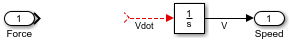3. Model the drag force. Add a MATLAB Function block from the User-Defined Functions library. The MATLAB Function block provides a quick way to implement mathematical expressions in your model. To edit the function, double-click the block to open the MATLAB Function editor.4. Define arguments for the MATLAB Function block. In the MATLAB Function block editor, click the Edit Databutton. Click k_drag, set Scope to Parameter, and click Apply.

5. Subtract the drag force from the motor force with Subtract block. Complete the force-acceleration equation with a Gain block with parameter 1/(m/2).

6. To reverse the direction of the MATLAB Function block, right-click the block and select Rotate & Flip > Flip Block. Connect the blocks.7. The dynamics of the two wheels are the same. Make a copy of the Right Wheel subsystem you just modeled and paste it in the Left Wheel subsystem.

8. View the top level of the model. Click the button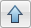.

Rotational Motion.   When the two wheels turn in opposite directions, they move in a circle of radius r, causing rotational motion of the robot. When the wheels turn in the same direction, there is no rotation. Assuming that the wheel velocities are always equal in magnitude, it is practical to model rotational motion as dependent on the difference of the two wheel velocities VR and VL:

$\stackrel{˙}{\theta }=\frac{{V}_{R}-{V}_{L}}{2r}$

Build the rotation dynamics model:

1. In the top level of the system_layout model, double-click the Rotation subsystem to display the empty subsystem. Delete the connection between the Inport and the Outport blocks.

2. Model angular speed and angle. Add an Integrator block. Leave the initial condition set to 0. The output of this block is the angle theta and the input is the angular speed theta_dot.

3. Compute angular speed from tangential speed. Add a Gain with parameter 1/(2*r).

4. Connect the blocks.5. View the top level of the model. Click the button.

Model the Functional Components

Describe the function from the input of a function to its output. This description can include algebraic equations and logical constructs, which you can use to build a graphical model of the system in Simulink.

Coordinate Transformation.  The velocity of the robot in the X and Y coordinates, VX and VY, is related to the linear speed VN and the angle theta:

$\begin{array}{l}{V}_{X}=-{V}_{N}\mathrm{cos}\left(\theta \right)\\ {V}_{Y}={V}_{N}\mathrm{sin}\left(\theta \right)\end{array}$

Build the coordinate transformation model:

1. In the top level of the system_layout model, double-click the Coordinate Transform subsystem to display the empty subsystem.

2. Model trigonometric functions. Add a SinCos block from the Math Operations library.

3. Model multiplications. Add two Product blocks from the Math Operations library.

4. Connect the blocks.5. View the top level of the model. Click the button.

Set Model Parameters

A source for model parameter values can be:

• Written specifications such as standard property tables or manufacturer data sheets

• Direct measurements on an existing system

• Estimations using system input/output

This model uses these parameters:

ParameterSymbolValue
Massm2.5 kg
Rolling resistancek_drag30 Ns2/m

Simulink uses the MATLAB® workspace to evaluate parameters. Set these parameters in the MATLAB command window:

m = 2.5;
k_drag = 30;
r = 0.15;

Validate Components Using Simulation

Validate components by supplying an input and observing the output. Even such a simple validation can point out immediate ways to improve the model. This example validates these behaviors:

• When a force is applied continuously to a wheel, the velocity increases until it reaches a steady-state velocity.

• When the wheels turn in opposite directions, the rotation angle increases at a constant rate.

Validate Wheel Component

Create and run a test model for the wheel component:

1. Create a new model. In the Simulation tab, click New. Copy the Right Wheel block into the new model.

2. Create a test input. Add a Step block from the Sources library and connect it to the input of the Right Wheel block. Leave the step time parameter set to 1.

3. Add a viewer to the output. Right-click the output port of the Right Wheel block and select Create & Connect Viewer > Simulink > Scope.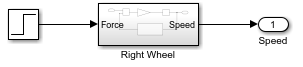4. Run the simulation. In the Simulation tab, click Run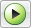.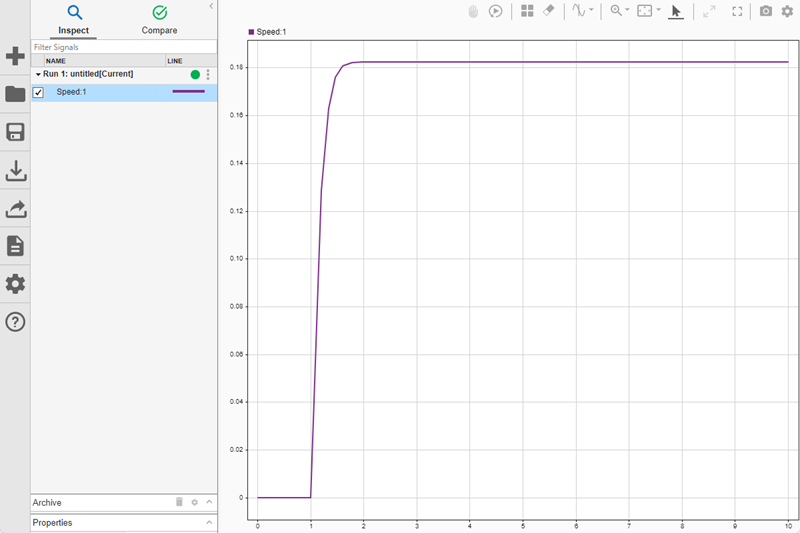The simulation result exhibits the general expected behavior. There is no motion until force is applied at step time. When force is applied, the speed starts increasing and then settles at a constant when the applied force and the drag force reach an equilibrium. In addition to validation, this simulation also gives information on the maximum speed of the wheel for the given force.

Validate Rotation Component

Create and run a test model for the rotation model:

1. Create a new model. Clickand copy the Rotation block into the new model.

2. Create a test input in the new model. Add a Step block from the Sources library. Leave the step time parameter set to 1. Connect it to the input of the Rotation block. This input represents the difference of the wheel velocities when the wheels are rotating in opposite directions.

3. Add a viewer to the output. Right-click the output port of the Rotation block and select Create & Connect Viewer > Simulink > Scope.4. Run the simulation. In the Simulation tab, click Run.This simulation shows that the angle increases steadily when the wheels are turning with the same speed in opposite directions. You can make some model improvements to make it easier to interpret the angle output, for example:

• You can convert the output in radians to degrees. Add a Gain block with a gain of 180/pi.

• You can display the degrees output in cycles of 360 degrees. Add a Math Function block with function mod.

MATLAB trigonometric functions take inputs in radians.

Validate the Model

After you validate individual components, you can perform a similar validation on the complete model. This example validates the following behavior:

• When the same force is applied to both wheels in the same direction, the robot moves in a line.

• When the same force is applied to both wheels in opposite directions, the robot rotates in place.

1. In the system_layout model, double-click the Inputs subsystem to display the empty subsystem.

2. Create a test input by adding a Step block. Leave the step time parameter set to 1. Connect it to both Outport blocks.3. At the top level of the model, connect both output signals to the same scope viewer: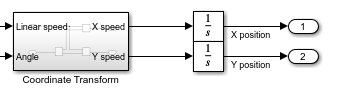4. Run the model.In this figure, the yellow line is the X direction and the blue line is the Y direction. Since the angle is zero and is not changing, the vehicle moves only in the X direction, as expected.

5. Double-click the Inputs subsystem and add a Gain with parameter -1 between the source and the second output. This reverses the direction for the left wheel.6. Add a scope to the angle output.

7. Run the model.The first view shows that there is no motion in the X-Y plane. The second view shows that there is steady rotation.

You can use this final model to answer many questions about the model by changing the input. Some examples are:

• What happens when the initial angle is not zero?

• How long does it take for the motion to stop when the force drops to zero?

• What happens when the robot is heavier?

• What happens when the robot moves on a smoother surface, that is, when the drag coefficient is lower?